RRB JE IT (CBT I) Mock Test- 1

# RRB JE IT (CBT I) Mock Test- 1

Test Description

## 100 Questions MCQ Test RRB JE Mock Test Series for Computer Science Engineering | RRB JE IT (CBT I) Mock Test- 1

RRB JE IT (CBT I) Mock Test- 1 for Railways 2023 is part of RRB JE Mock Test Series for Computer Science Engineering preparation. The RRB JE IT (CBT I) Mock Test- 1 questions and answers have been prepared according to the Railways exam syllabus.The RRB JE IT (CBT I) Mock Test- 1 MCQs are made for Railways 2023 Exam. Find important definitions, questions, notes, meanings, examples, exercises, MCQs and online tests for RRB JE IT (CBT I) Mock Test- 1 below.
Solutions of RRB JE IT (CBT I) Mock Test- 1 questions in English are available as part of our RRB JE Mock Test Series for Computer Science Engineering for Railways & RRB JE IT (CBT I) Mock Test- 1 solutions in Hindi for RRB JE Mock Test Series for Computer Science Engineering course. Download more important topics, notes, lectures and mock test series for Railways Exam by signing up for free. Attempt RRB JE IT (CBT I) Mock Test- 1 | 100 questions in 90 minutes | Mock test for Railways preparation | Free important questions MCQ to study RRB JE Mock Test Series for Computer Science Engineering for Railways Exam | Download free PDF with solutions
 1 Crore+ students have signed up on EduRev. Have you?
RRB JE IT (CBT I) Mock Test- 1 - Question 1

### A student who gets 30% of the marks in an exam fails by 50 marks. Another student who gets 320 marks fails by 30 marks. Find the maximum marks.

Detailed Solution for RRB JE IT (CBT I) Mock Test- 1 - Question 1

Let m as maximum marks

Now, according to question

⇒ 0.3 m + 50=320 + 30

⇒0.3 m = 300

m = 1000 marks

RRB JE IT (CBT I) Mock Test- 1 - Question 2

### If the roots of the equation 6x2 - 25x + p = 0 are real and different, then what can be the value of 'p' if the value of p is any integer greater than 18?

Detailed Solution for RRB JE IT (CBT I) Mock Test- 1 - Question 2

Since, the roots of equation 6x2 - 25x + p = 0 are real and different. So, its discriminant should be positive.

b2 - 4ac > 0

b2 > 4ac

(-25)2 > 4 * 6 * p

625 > 24p

p < 625/24

p < 26.04

Since the value of p is any integer greater than 18 and less than 26.

So, the possible values of p = 19, 20, 21, 22, 23, 24, 25 and 26.

RRB JE IT (CBT I) Mock Test- 1 - Question 3

### A trader sells two bullocks for Rs 9100 each, neither losing nor gaining in total. If he sold one of the bullocks at a gain of 30%, the other is sold at a loss of

Detailed Solution for RRB JE IT (CBT I) Mock Test- 1 - Question 3

The total selling price of two bullocks = 9100*2 = Rs 18200

Cost price of 1st bullock = 9100*(100/130) = 7000

According to the question, there is no profit or loss.

Therefore cost price of second bullock = 18200 - 7000 = Rs 11200

Selling price of second bullock = Rs 9100

Therefore loss = 11200 - 9100 = Rs 2100

Hence % loss on second bullock = (2100/11200)*100 = 18.75%

RRB JE IT (CBT I) Mock Test- 1 - Question 4

Two boxes have the same height, equal to 35 cm. One box has a square base of 10 cm. The other box is cylindrical, and the diameter of its base is 12 cm. What is the difference in their volumes?

Detailed Solution for RRB JE IT (CBT I) Mock Test- 1 - Question 4

Required Difference = Volume of cylinder - volume of cuboid

= πr2h - lbh

= 22/7 x 6 x 6 x 35 - 35 x 10 x 10

= 3960 - 3500 = 460 cm3

RRB JE IT (CBT I) Mock Test- 1 - Question 5

3 men can complete a piece of work in 6 days. 5 women can complete the same work in 18 days. In how many days will 4 men and 10 women together complete the same work?

Detailed Solution for RRB JE IT (CBT I) Mock Test- 1 - Question 5

3x6 men = 5x18 women

⇒ 1 man = 5 women

4 men + 10 women = 6 men

M1D1 = M2D2

⇒ 3x6 = 6D2

⇒ D2 = 3 days

RRB JE IT (CBT I) Mock Test- 1 - Question 6

What is the equation of line of slope 0.8 and intercept at y-axis is 0.5?

Detailed Solution for RRB JE IT (CBT I) Mock Test- 1 - Question 6

Equation of line-

y = mx + c

Where, m = slope and c = y intercept

Required equation of line-

y = (0.8)x + (0.5)

10y = 8x + 5

8x - 10y + 5 = 0

RRB JE IT (CBT I) Mock Test- 1 - Question 7

If there are 15 numbers in the given arithmetic series, then what will be the last term?

293, 305, 317,......

Detailed Solution for RRB JE IT (CBT I) Mock Test- 1 - Question 7

The following series is in the arithmetic progression, as the difference between every two consecutive terms is same. So,

According to the question:

a = 293

d = 305 - 293 = 12

n = 15

an = a + (n - 1)d

= 293 + 14 x 12

= 461

RRB JE IT (CBT I) Mock Test- 1 - Question 8

A faulty watch loses 3 minutes every 25 minutes. It was set right at 11:24 p.m. If, on the next day, it shows 01:36 a.m. on the watch, what is the actual time?

Detailed Solution for RRB JE IT (CBT I) Mock Test- 1 - Question 8

Duration from 11:24 p.m. to 01:36 a.m. = 2 hours 12 minutes = 132 minutes.

22 minutes in faulty watch = 25 minutes of actual time

⇒ 22*(132/22) minutes in faulty watch = 25*(132/22) minutes of actual time

⇒ 132 minutes in faulty watch = 150 minutes of actual time or 2 hours 30 minutes of actual time.

Actual time is 11:24 p.m. + 2 hours 30 minutes = 01:54 a.m.

RRB JE IT (CBT I) Mock Test- 1 - Question 9

If the height of an equilateral triangle is 2√3 cm, then what is the length of the circumradius of that triangle?

Detailed Solution for RRB JE IT (CBT I) Mock Test- 1 - Question 9

The Left side of the equilateral triangle is 'a' cm.

Height of equilateral triangle = √[a2 - (a/2)2] = (a√3)/2 = 2√3

a = 4 cm

Area of triangle = (1/2) * side * Height = (1/2) * 4 * 2√3 = 4√3 cm2

Length of circumradius of triangle = abc/4△

= (4 * 4 * 4)/(4 * 4√3)

= (4/√3) cm

RRB JE IT (CBT I) Mock Test- 1 - Question 10

87.5% of a number exceeds 32.5% of it by 220. Then 28.5% of the number is

Detailed Solution for RRB JE IT (CBT I) Mock Test- 1 - Question 10

Let the no. be N.

Then 87.5% of N - 32.5% of N = 220

⇒ 55% of N = 220

⇒ N = 400

28.5% of 400 = 114

RRB JE IT (CBT I) Mock Test- 1 - Question 11

The ratio of the ages of the father and the son at present is 4:1. 4 years later, the ratio will be 3:1. What was the ratio of their ages 4 years ago?

Detailed Solution for RRB JE IT (CBT I) Mock Test- 1 - Question 11

Present age: father =4x son =x

Now, (4x+4)/(x+4) = 3/1

⇒ X= 8.

4 years ago the ages were: father= 32-4=28 son=8-4=4

The required ratio = 28:4 = 7:1

RRB JE IT (CBT I) Mock Test- 1 - Question 12

Find the sum to infinity of the geometric progression.

2/3, 2/9, 2/27, 2/81......

Detailed Solution for RRB JE IT (CBT I) Mock Test- 1 - Question 12

The given geometric progression: 2/3, 2/9, 2/27, 2/81......

a = 2/3

Common ratio, r = (2/9)/(2/3) = 1/3 < 1

Therefore, the sum of infinity is given by:

Sum = a/(1 - r) = (2/3)/(1 - 1/3) = 1

RRB JE IT (CBT I) Mock Test- 1 - Question 13

What value we get when: (123 - 54 + 22) is divided by (112 + 2)?

Detailed Solution for RRB JE IT (CBT I) Mock Test- 1 - Question 13

= (123 - 54 + 22) ÷ (112 + 2)

= (1728 - 625 + 4) ÷ (123)

= (1107) * (1/123)

= 9

= 32

RRB JE IT (CBT I) Mock Test- 1 - Question 14

What is the value of (34 x 289 ÷ 17 + 23 x 22) ÷ (18 x 88 - 79 x 20)?

Detailed Solution for RRB JE IT (CBT I) Mock Test- 1 - Question 14

= (34 x 289 ÷ 17 + 23 x 22) ÷ (18 x 88 - 79 x 20)

= (578 + 506) ÷ (1584 - 1580)

= 1084 ÷ 4

= 271

RRB JE IT (CBT I) Mock Test- 1 - Question 15

Seven times the denominator of the fraction is five more than nine times the numerator, and ten times the numerator is eight less than eight times the denominator. Find out the reciprocal of the fraction.

Detailed Solution for RRB JE IT (CBT I) Mock Test- 1 - Question 15

Let us assume the fraction as N/D.

So, 7D = 9N + 5 .... (1)

And, 10N = 8D - 8 .... (2)

Solving these two equations we get,

D = 11 and N = 8.

Since we need to find the reciprocal, so the answer is 11/8.

RRB JE IT (CBT I) Mock Test- 1 - Question 16

Express 6.121212.. into (p/q) form.

Detailed Solution for RRB JE IT (CBT I) Mock Test- 1 - Question 16

Let X = 6.121212.. (I)

⇒ 100X = 612.121212.. (II)

(II) - (I) gives,

99X = 606

⇒ X = 202/33

RRB JE IT (CBT I) Mock Test- 1 - Question 17

What will be the sum of compound interest and simple interest on Rs 25000 at 20% per annum for 2(1/4) years?

Detailed Solution for RRB JE IT (CBT I) Mock Test- 1 - Question 17

The amount after 2(1/4) years

= 25000 * (1 + 20/100)2(1+(20/4)/100)

= Rs 37800

So compound interest= Rs (37800 - 25000) = Rs 12800

Simple interest= (25000 * 20 * (9/4))/100= Rs 11250

Sum of compound and simple interest= Rs (12800 + 11250) = Rs 24050

RRB JE IT (CBT I) Mock Test- 1 - Question 18

A Clock shows 7 a.m. Through how many degrees has the hour hand-turned by 1 p.m. on the same day?

Detailed Solution for RRB JE IT (CBT I) Mock Test- 1 - Question 18

7 a.m. is directly opposite of 1 p.m.

Angle travelled by hour hand = 180°

Alternate method:

number of hours between 7a.m. and 1p.m. = 6

degrees travelled by hour hand in 1 hour = 30o

required answer = 6 X 30 = 180o

RRB JE IT (CBT I) Mock Test- 1 - Question 19

What is that minimum number which, when divided by 9, 11 and 15, leaves remainders 4, 6 and 10 respectively?

Detailed Solution for RRB JE IT (CBT I) Mock Test- 1 - Question 19

Here k = (9 - 4) = (11 - 6) = (15 - 10) = 5

The least number which when divided by 9, 11 and 15 leaves remainders 4, 6 and 10 respectively = (LCM of 9, 11 and 15) - k

LCM of 9, 11 and 15 = 495

Required number = 495 - 5 = 490

RRB JE IT (CBT I) Mock Test- 1 - Question 20

Following Bar graph shows the marks obtained by Sita and Gita in 5 Subjects: English, Hindi, Mathematics, Geography and history(all out of 100).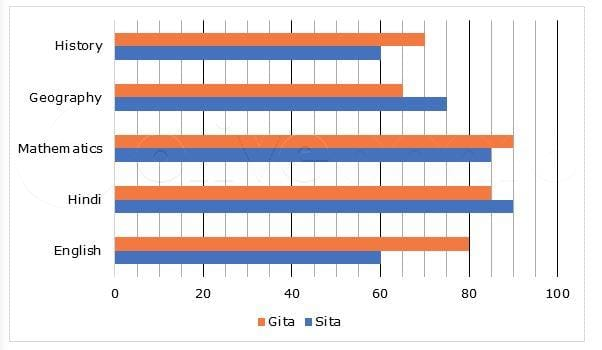What is the difference between the average marks obtained by Gita and Sita?

Detailed Solution for RRB JE IT (CBT I) Mock Test- 1 - Question 20

Difference between the average marks obtained by Sita and Gita

= (70 + 65 + 90 + 85 + 80)/5 - (60 + 75 + 85 + 90 + 60)/5 = 4

RRB JE IT (CBT I) Mock Test- 1 - Question 21

What is the digit in the unit place of 251?

Detailed Solution for RRB JE IT (CBT I) Mock Test- 1 - Question 21

The digit in the unit's place of 251 is equal to the remainder when 251 is divided by 10.

25 =32 leaves the remainder 2 when divided by 10.

Then 250 = (25)10 leaves the remainder 210 =(25)2 which in turn leaves the remainder 22= 4

Then 251= 250x 2, when divided by 10, leaves the remainder 4 x 2=8

RRB JE IT (CBT I) Mock Test- 1 - Question 22

What will we get on factorization of (x4 + 6x3 + 7x2 - 6x - 8)?

Detailed Solution for RRB JE IT (CBT I) Mock Test- 1 - Question 22

F(x) = x4 + 6x3 + 7x2 - 6x - 8

By hit and trial method, we get -

x = 1, gives F (1) = 0, so, (x - 1) is a factor of F (x)

F (1) = 1 + 6 + 7 - 6 - 8 = 0

Similarly, F(-1)= 0 = 1 + 6(-1) + 7 - 6(-1) - 8 = 1 - 6 + 7 + 6 - 8 = 0

Hence,

(x - 1)(x + 1) i.e. (x2 - 1) is a factor of F (x)

On dividing F(x) by (x2 - 1), we get,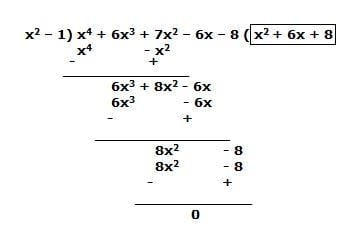Hence, (x2 + 6x + 8) is other factor = (x+2)(x+4)

Thus, option (c) is correct.

RRB JE IT (CBT I) Mock Test- 1 - Question 23

The average of the first 10 prime numbers is

Detailed Solution for RRB JE IT (CBT I) Mock Test- 1 - Question 23

First 10 prime numbers are 2, 3, 5, 7, 11, 13, 17, 19, 23, 29

Hence, average of all these numbers = (2+3+5+7+11+13+17+19+23+29)/10 = 129/10 = 12.9

RRB JE IT (CBT I) Mock Test- 1 - Question 24

When the place value of 7 in '16274529' is divided by the place value of 3 in '672345' then, what is the remainder obtained?

Detailed Solution for RRB JE IT (CBT I) Mock Test- 1 - Question 24

Place value of a digit in any number = (face value of the digit) *(value of the place at which digit occurs)

Place value of 7 in '16274529' = 7*10000= 70000

Place value of 3 in '672345' = 300

70000/300 = 700/3

Remainder = 1

RRB JE IT (CBT I) Mock Test- 1 - Question 25

30 litres of milk is mixed with 15 litres of water to form a mixture; after this, 30 litres of this mixture is mixed with 10 litres of water to form another mixture. Now, 16 litres of this new mixture is mixed with 10 litres of pure milk and 4 litres of water to form the final mixture. What is the percent profit earned after selling the final mixture?

Given below are the steps involved. Arrange them in sequential order.

(a) Ratio of milk to water when 30 litres of milk is mixed with 15 litres of water = 30: 15 = 2: 1

(b) Percent profit = (12/18) * 100 = 66(2/3)%

(c) Ratio of milk to water when 16 litres of the mixture is mixed with 10 litres of pure milk and 4 litres of water = [16 * (1/2) + 10]: [16 * (1/2) + 4] = 18: 12 = 3: 2

(d) Ratio of milk to water when 30 litres of the mixture is mixed with 10 litres of water = [30 * (2/3)]: [30 * (1/3) + 10] = 20: 20 = 1: 1

(E) Amount of milk and water in the final mixture is 18 litres and 12 litres, respectively.

Detailed Solution for RRB JE IT (CBT I) Mock Test- 1 - Question 25

The correct order is:

(a) Ratio of milk to water when 30 litres of milk is mixed with 15 litres of water = 30: 15 = 2: 1

(d) Ratio of milk to water when 30 litres of the mixture is mixed with 10 litres of water = [30 * (2/3)]: [30 * (1/3) + 10] = 20: 20 = 1: 1

(c) Ratio of milk to water when 16 litres of the mixture is mixed with 10 litres of pure milk and 4 litres of water = [16 * (1/2) + 10]: [16 * (1/2) + 4] = 18: 12 = 3: 2

(E) Amount of milk and water in the final mixture is 18 litres and 12 litres, respectively.

(b) Percent profit = (12/18) * 100 = 66(2/3)%

RRB JE IT (CBT I) Mock Test- 1 - Question 26

What is the standard deviation of numbers given below?

5, 8, 9, 12, 12, 10, 16, 8

Detailed Solution for RRB JE IT (CBT I) Mock Test- 1 - Question 26

Mean = (5 + 8 + 9 + 12 + 12 + 10 + 16 + 8)/8 = 10

Variance = ((5 - 10)2 + (8 - 10)2 + (9 - 10)2 + (12 - 10)2 + (12 - 10)2 + (10 - 10)2 + (16 - 10)2 + (8 - 10)2)/8

= 39/4

Standard deviation = √variance = √(39/4) = √39/2

RRB JE IT (CBT I) Mock Test- 1 - Question 27

The sum and difference of the LCM and the HCF of the two numbers are 624 and 546 respectively. If the sum of two numbers is 312, find the numbers.

Detailed Solution for RRB JE IT (CBT I) Mock Test- 1 - Question 27

Let the LCM and HCF are p and q, respectively.

So, p + q = 624, p - q = 546

Therefore, p = (624+546)/2 = 585 and q = (624-546)/2 = 39

That means LCM = 585 and HCF = 39

Now, let the numbers be 39a and 39b, where a and b are co-primes.

Therefore 39a + 39b = 312 => a + b = 312/39 = 8

So possible pairs of co-primes, whose sum is 8, are (3,5) and (1,7).

Therefore possible pairs of numbers are

(39*3, 39*5) = (117,195) and

(39*1, 39*7) = (39,273)

Now, LCM*HCF = 585*39 = 22815

Also, 117*195 = 22815 and 39*273 = 10647

Hence, the required numbers are 117 and 195 because the product of two numbers is equal to the product of their LCM and HCF.

RRB JE IT (CBT I) Mock Test- 1 - Question 28

Which of the following pairs of numbers are needed to be interchanged to make LHS = RHS?.

6 + 5 x 4 - 10 x 2 = 9 x 3 - 7 x 5 + 12

Detailed Solution for RRB JE IT (CBT I) Mock Test- 1 - Question 28

After replacing 10 and 12, we get: -

6 + 5 x 4 - 12 x 2 = 9 x 3 - 7 x 5 + 10

6 + 20 - 24 = 27 - 35 + 10

2 = 2.

So, option d is the correct answer.

RRB JE IT (CBT I) Mock Test- 1 - Question 29

If it was Sunday on 1st July 2012, then which day is 18th November 2012?

Detailed Solution for RRB JE IT (CBT I) Mock Test- 1 - Question 29

Number of days from 1st July to 1st November = 31+ 31 + 30 + 31= 123

Remainder days = 123 - 119= 4 (119 is divisible by 7)

So,

November 1st 2012= Sunday + 4= Thursday

Thus, 15th November = 1+14= Thursday

18th November = Thursday + 3 = Sunday

RRB JE IT (CBT I) Mock Test- 1 - Question 30

A person reaches his office 5 minutes late if he travels at 60 km/h and is 10 minutes early if the speed is 90 km/h. Find the distance he needs to travel.

Detailed Solution for RRB JE IT (CBT I) Mock Test- 1 - Question 30

Speed ratio = 60 : 90 = 2 : 3

So, time ratio = 3 : 2

So we can assume the time as 3x and 2x.

Hence 3x - 2x = 10 + 5

Since here he is 5 minutes late and 10 minutes early, so the difference is the summation.

So 2x = 30 minutes.

Hence with a speed of 90 km/h, it takes 30 minutes, so the distance is 45 km.

RRB JE IT (CBT I) Mock Test- 1 - Question 31

D said A’s father is the only brother of my sister’s son. How is A’s father related to D?

Detailed Solution for RRB JE IT (CBT I) Mock Test- 1 - Question 31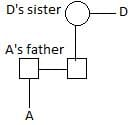A’s father is the nephew of D. Hence option (b) is correct.

RRB JE IT (CBT I) Mock Test- 1 - Question 32

In the given sequence, how many such symbols and numbers are there, which are either immediately preceded or immediately followed by the letters which are from the first half of the English alphabet?

2 L K @ 8 \$ P B 1 V # 6 % G W 9 J C D 4 © 7 F R 4 A

Detailed Solution for RRB JE IT (CBT I) Mock Test- 1 - Question 32 Such combinations are

2L, K@, B1, %G, 9J, D4, 7F, 4A,

RRB JE IT (CBT I) Mock Test- 1 - Question 33

In the following questions, find out the answer figure which completes the problem figure matrix.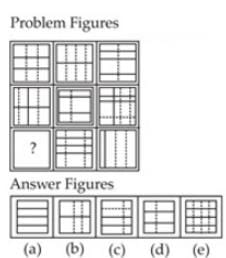Detailed Solution for RRB JE IT (CBT I) Mock Test- 1 - Question 33 Each row and column contains six complete lines and six broken lines.
RRB JE IT (CBT I) Mock Test- 1 - Question 34

Study the following diagram and answer the questions that follow.

a. The rectangle represents men.

c. Triangle represents skilled persons.

d. Square represents employed persons.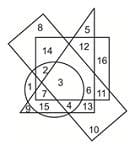The skilled employed women who are graduates are-

RRB JE IT (CBT I) Mock Test- 1 - Question 35

In the questions given below, some relationship has been expressed through symbols as shown below. Based on the meaning of these symbols and choose the correct answer.

ϕ means ‘less than.’

Δ means ‘not greater than.’

- means ‘equal to.’

+ means ‘not equal to.’

× means ‘not less than.’

= means ‘greater than.’

X - Y = Z implies

Detailed Solution for RRB JE IT (CBT I) Mock Test- 1 - Question 35

Clearly, the meaning of given symbols

X - Y = Z ⇒ X = Y > Z

Using the proper notations/symbols in option(b), we get

X Δ Y x Z ⇒ X ≤ Y ≥ Z ⇒ X = Y > Z

Therefore, X - Y = Z ⇒ X Δ Y x Z

RRB JE IT (CBT I) Mock Test- 1 - Question 36

Find the missing term.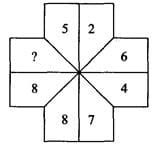Detailed Solution for RRB JE IT (CBT I) Mock Test- 1 - Question 36

There are two alternate series.

1st series: 5, 6, 7, 8, 9, …... and so on.

2nd series: 2 (×2), 4 (×2), 8 (×2) ... and so on.

RRB JE IT (CBT I) Mock Test- 1 - Question 37

How many hexagons are there in the figure given below?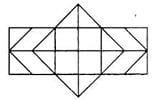Detailed Solution for RRB JE IT (CBT I) Mock Test- 1 - Question 37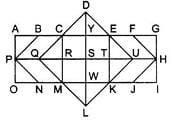∴ Total hexagons= CDEKLM, CEUKMQ, CFHJMQ, BEUKNP, BFHJNP = 5

RRB JE IT (CBT I) Mock Test- 1 - Question 38

‘Suma’ is shorter than ‘Uma’, ‘Neha is taller than ‘Suma’, ‘Sudha’ is taller than ‘Uma’ but shorter than ‘Hema’. ‘Uma’ is taller than ‘Neha’. Who is the tallest among them?

Detailed Solution for RRB JE IT (CBT I) Mock Test- 1 - Question 38

Uma > Suma ---------------- (i)

Neha > Suma ------------------(ii)

Hema > Sudha > Uma > Neha ---------(iii)

On combining the above statement,

Hema > Sudha > Uma > Neha > Suma

Therefore, Hema is the tallest among all.

RRB JE IT (CBT I) Mock Test- 1 - Question 39

A word is represented by only one set of numbers as given in anyone of the alternatives. The set of numbers given in the alternatives are represented by two classes of alphabets as in the 2 matrices given below. The columns and rows of Matrix I are from 0 to 4 and that of Matrix II from 5 to 9. A letter can be represented first by its row and next by its column number. e.g., ‘C’ can be represented by 02, 21, etc. ‘T’ can be represented by 65, 96 etc. Similarly, you have to identify the set of words for ‘STAMP’.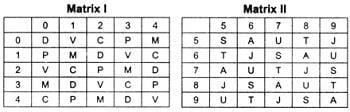Detailed Solution for RRB JE IT (CBT I) Mock Test- 1 - Question 39 According to the matrices,

S = 55, 86. 67, 98, 79

T = 65, 96, 77, 58, 89

A = 75, 56, 87, 68, 99

M = 30, 11, 42, 23, 04

P = 10, 41, 22, 03, 34

∴ Stamp = 98, 89, 75, 23, 34

RRB JE IT (CBT I) Mock Test- 1 - Question 40

Choose the odd one out of the given alternatives.

Detailed Solution for RRB JE IT (CBT I) Mock Test- 1 - Question 40 In all other groups, there is a gap of one letter as in the alphabet between the first and third letter.
RRB JE IT (CBT I) Mock Test- 1 - Question 41

DRIVEN is related to EIDRVN in the same way as BEGUM is related to ________.

Detailed Solution for RRB JE IT (CBT I) Mock Test- 1 - Question 41

As,

1 2 3 4 5 6

D R I V E N

E I D R V N

5 3 1 2 4 6

Similarly,

1 2 3 4 5

B E G U M

M G B E U

5 3 1 2 4

RRB JE IT (CBT I) Mock Test- 1 - Question 42

Choose the odd one out from the given alternatives.

Detailed Solution for RRB JE IT (CBT I) Mock Test- 1 - Question 42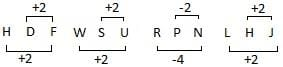RRB JE IT (CBT I) Mock Test- 1 - Question 43

Select the related word from the given alternatives.

BCG: URP:: LMQ: ???

Detailed Solution for RRB JE IT (CBT I) Mock Test- 1 - Question 43 The series will be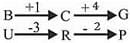Same way,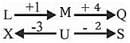RRB JE IT (CBT I) Mock Test- 1 - Question 44

If HOSPITAL is written as 32574618 in a certain code, how would POSTAL be written in that code?

Detailed Solution for RRB JE IT (CBT I) Mock Test- 1 - Question 44

H O S P I T A L

↓ ↓ ↓ ↓ ↓ ↓ ↓ ↓

3 2 5 7 4 6 1 8

So,

P O S T A L

↓ ↓ ↓ ↓ ↓ ↓

7 2 5 6 1 8

RRB JE IT (CBT I) Mock Test- 1 - Question 45

Three of the following four are alike in a certain way and hence form a group. Which is the one that does not belong to that group?

Detailed Solution for RRB JE IT (CBT I) Mock Test- 1 - Question 45

Option (a):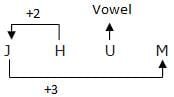Option (b):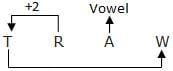Option (c):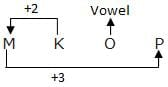Option (d):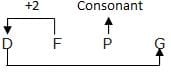Here option (d) does not belong to the group.

RRB JE IT (CBT I) Mock Test- 1 - Question 46

In the question below, there are few statements followed by few conclusions. You have to take the given statements to be true even if they seem to be at variance with commonly known facts and then decide which of the given conclusions logically follow(s) from the given statements.

Statements:

1. Some books are pens.

2. Some pens are pencils.

3. All pencils are rubber.

Conclusions:

I. Some books are pencils.

II. No book is a pencil.

Detailed Solution for RRB JE IT (CBT I) Mock Test- 1 - Question 46 Either conclusion I or II follows.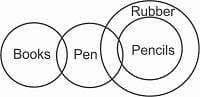RRB JE IT (CBT I) Mock Test- 1 - Question 47

Identify the diagram that best represents the relationship among Iron, Lead, Nitrogen.

Choose the correct Venn diagram.

Detailed Solution for RRB JE IT (CBT I) Mock Test- 1 - Question 47 There is no relation between iron, lead and nitrogen.

So,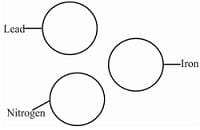RRB JE IT (CBT I) Mock Test- 1 - Question 48

In the following questions, a word is followed by five other words, one of which cannot be formed by using the letters of the given word. Find this word

CLASSIFICATION

Detailed Solution for RRB JE IT (CBT I) Mock Test- 1 - Question 48 As there is only one ‘N’ letter in the given word
RRB JE IT (CBT I) Mock Test- 1 - Question 49

If in a certain code language ‘CONTROL’ is written as ‘DMQPWIS’, then how will ‘BROTHQR’ be written in that code?

Detailed Solution for RRB JE IT (CBT I) Mock Test- 1 - Question 49

3 15 14 20 18 15 12

C O N T R O L

↓+1 ↓-2 ↓+3 ↓-4 ↓+5 ↓-6 ↓+7

D M Q P W I S

4 13 17 16 23 9 19

Similarly,

2 18 15 20 8 17 18

B R O T H Q R

↓+1 ↓-2 ↓+3 ↓-4 ↓+5 ↓-6 ↓+7

C P R P M K Y

3 16 18 16 13 11 25

RRB JE IT (CBT I) Mock Test- 1 - Question 50

Mohan started walking East and walked 30 metres, then he turned right and walked 50 metres, and he again turned left and walked 40 metres. Again he turned left and walked 50 metres. Now, how far is he from his starting point?

Detailed Solution for RRB JE IT (CBT I) Mock Test- 1 - Question 50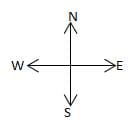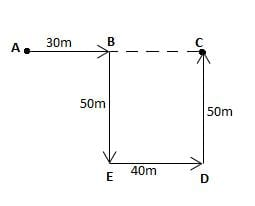Start point is A

End point is C

AC = AB + BC

= 30 + 40

= 70 (BC = ED)

RRB JE IT (CBT I) Mock Test- 1 - Question 51

Solve the following series?

B2F, G4K, L12P, Q38U, ?

Detailed Solution for RRB JE IT (CBT I) Mock Test- 1 - Question 51

The series is as follows: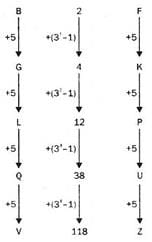V118Z will come in place of ‘?’.

RRB JE IT (CBT I) Mock Test- 1 - Question 52

Find the odd one out from the given alternatives.

Detailed Solution for RRB JE IT (CBT I) Mock Test- 1 - Question 52 In all other groups, there is a gap of two letters as in the alphabet between the third and fourth letter.
RRB JE IT (CBT I) Mock Test- 1 - Question 53

What should come at the place of the question mark?

2, A, 9, B, 6, C, 13, D,?

Detailed Solution for RRB JE IT (CBT I) Mock Test- 1 - Question 53

Here,

Series I = 2, 9, 6, 13, ?

Series II = A, B, C, D

n Series I,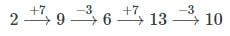RRB JE IT (CBT I) Mock Test- 1 - Question 54

A and B are sisters. R and S are brothers. A’s daughter is R’s sister. What is B’s relation to S?

Detailed Solution for RRB JE IT (CBT I) Mock Test- 1 - Question 54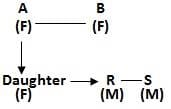Clearly, B is the aunt of S.

RRB JE IT (CBT I) Mock Test- 1 - Question 55

There are two sets of figures, namely problem Figure containing five figures 1, 2, 3, 4, 5 and answer figures (a), (b), (c), (d). You have to select one Figure from the answer set, which will continue the same series as given in problem set figures.

Problem figure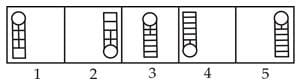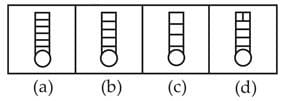Detailed Solution for RRB JE IT (CBT I) Mock Test- 1 - Question 55 Problem figure 2 is the reverse of figure 1 with the same number of horizontal lines and vertical lines decreases by one row. Similarly, Figure 4 is the reverse of figure 3 and symmetrical changes in the pattern of lines. Therefore, answer figure (a) is the reverse of problem figure 5.
RRB JE IT (CBT I) Mock Test- 1 - Question 56

When was Delhi annexed by the Britishers for the first time?

Detailed Solution for RRB JE IT (CBT I) Mock Test- 1 - Question 56 After the second Anglo-Maratha war, the then powerful Maratha Confederacy was defeated. And it ensured that no reasonable power was capable of having control over Delhi. And for the first time, it was annexed by Lord Lacke in 1803.
RRB JE IT (CBT I) Mock Test- 1 - Question 57

About 50% of the world population is concentrated between the latitudes of_____?

Detailed Solution for RRB JE IT (CBT I) Mock Test- 1 - Question 57 Most populous countries like China, India, the USA and North African countries are situated between 20° N and 40° N.
RRB JE IT (CBT I) Mock Test- 1 - Question 58

In which state of India the Thattekkad Bird Sanctuary is located?

Detailed Solution for RRB JE IT (CBT I) Mock Test- 1 - Question 58 The Thattekkad Bird Sanctuary, covering an area of barely 25 km2, and located about 12 km from Kothamangalam (Kerala state, India), was the first bird sanctuary in Kerala.
RRB JE IT (CBT I) Mock Test- 1 - Question 59

India is a secular state defined by____?

Detailed Solution for RRB JE IT (CBT I) Mock Test- 1 - Question 59 Secularism in India means equal treatment of all religions by the state. With the 42nd Amendment of the Constitution of India enacted in 1976, the Preamble to the Constitution asserted that India is a secular nation. However, neither India's constitution nor its laws define the relationship between religion and state.
RRB JE IT (CBT I) Mock Test- 1 - Question 60

What is the minimum age of Joining Atal Pension Yojana?

Detailed Solution for RRB JE IT (CBT I) Mock Test- 1 - Question 60 Minimum age of Joining Atal Pension Yojana is 18 years. APY was announced in the Finance Budget of 2015-16. The existing subscribers of the Swavalamban Scheme would be automatically migrated to APY unless they opt-out.
RRB JE IT (CBT I) Mock Test- 1 - Question 61

Kerala is famous for the cultivation of

1. Coconut 2. Black pepper 3. Rubber 4. Rice

Detailed Solution for RRB JE IT (CBT I) Mock Test- 1 - Question 61 Kerala is famous for the cultivation of coconut, tea, coffee, cashew and spices.
RRB JE IT (CBT I) Mock Test- 1 - Question 62

In the context of Indian agriculture, which irrigation system is most prominent in Andhra Pradesh?

Detailed Solution for RRB JE IT (CBT I) Mock Test- 1 - Question 62 Andhra Pradesh is leading in-tank irrigation with 28.8% of the total agricultural land of India, followed by Tamil Nadu.
RRB JE IT (CBT I) Mock Test- 1 - Question 63

During which dynasty, Mahabalipuram temple was constructed?

Detailed Solution for RRB JE IT (CBT I) Mock Test- 1 - Question 63 Mahabalipuram temple is also called The Shore Temple (built-in 700–728 AD). The Shore Temple is located in Mahabalipuram of Tamil Nadu. This temple is a fine specimen of Dravid architecture and is built with a block of granite. The Shore Temple is considered one of the oldest temples of South India, which is related to the eighth century. At the time of its creation, the site was a busy port during the reign of Narasimhavarman II of the Pallava dynasty. There are three temples within the Shore Temple. There is Lord Vishnu's temple in between; Shiva temple is on both sides of it.
RRB JE IT (CBT I) Mock Test- 1 - Question 64

Who formed the Satyagraha Sabha in Bombay?

Detailed Solution for RRB JE IT (CBT I) Mock Test- 1 - Question 64 In February 1919, Gandhiji founded the Satyagraha Sabha to protest against the Rowlatt Act.
RRB JE IT (CBT I) Mock Test- 1 - Question 65

What is the literal meaning of the term "Quo-Warranto"?

Detailed Solution for RRB JE IT (CBT I) Mock Test- 1 - Question 65 Quo Warranto: A legal proceeding during which an individual's right to hold any office or governmental privilege is challenged.
RRB JE IT (CBT I) Mock Test- 1 - Question 66

What will be the day of the week, 15th August 2010?

Detailed Solution for RRB JE IT (CBT I) Mock Test- 1 - Question 66

15th Aug 2010 = (2009 years + period from 1-Jan-2010 to 15-Aug-2010)

We know that number of odd days in 400 years = 0

Hence the number of odd days in 2000 years = 0 (Since 2000 is a perfect multiple of 400)

Number of odd days in the period 2001-2009 = 7normal years + 2leap year

= 7 x 1 + 2 x 2 = 11 = (11 - 7x1) odd day = 4 odd day

Days from1-Jan-2010 to15-Aug-2010 = 31 (Jan) + 28 (Feb) + 31 (Mar) + 30 (Apr) + 31(may) +

30(Jun) +31(Jul) + 15(Aug) = 227

227days = 32weeks + 3day =3 odd days

Total number of odd days = (0 + 4 + 3) = 7 odd days = 0 odd day

0 odd day = Sunday. Hence 15th August, 2010 is Sunday.

RRB JE IT (CBT I) Mock Test- 1 - Question 67

Which among the following amendments of the Constitution of India, Delhi was designated as National Capital Territory (NCT)?

Detailed Solution for RRB JE IT (CBT I) Mock Test- 1 - Question 67 According to article 239 of the Indian constitution, Union territory shall be administered by the President. Article 239AA of the Indian Constitution, enacted as per the 69th Amendment Act of 1991, confers special provisions for the National Capital Territory of Delhi.
RRB JE IT (CBT I) Mock Test- 1 - Question 68

Find the odd one out.

Detailed Solution for RRB JE IT (CBT I) Mock Test- 1 - Question 68 Except for option 3, the remaining are correct combinations. The Bahamas is a North American country.
RRB JE IT (CBT I) Mock Test- 1 - Question 69

The post of Vice President has been inspired by the constitution of

Detailed Solution for RRB JE IT (CBT I) Mock Test- 1 - Question 69 The Vice President shall be ex-officio chairman of the Rajya Sabha or the Council of States. The election of this post is conducted by the Election Commission, where an electoral college consisting of the members of both houses of parliament in accordance with the system of proportional representation can vote. The post has been inspired by the US constitution.
RRB JE IT (CBT I) Mock Test- 1 - Question 70

Which two countries’ Prime Ministers have been nominated for Nobel Peace Prize 2019 for signing the Prespa Agreement?

Detailed Solution for RRB JE IT (CBT I) Mock Test- 1 - Question 70 The Prime ministers of Macedonia and Greece, Zoran Zaev and Alexis Tsipras, are nominated for the 2019 Nobel Peace Prize for signing the Prespa Agreement between the two countries.
RRB JE IT (CBT I) Mock Test- 1 - Question 71

A resistor having a very low value of resistance is called ____________resistance.

Detailed Solution for RRB JE IT (CBT I) Mock Test- 1 - Question 71 Shunt Resistance has a very low value of resistance.

The shunt resistor is mainly made of the material having a low-temperature coefficient of resistance.

In an electrical circuit, it is generally connected in parallel with the ammeter whose range is to be extended.

RRB JE IT (CBT I) Mock Test- 1 - Question 72

Which of the following enzymes is present in the saliva of humans and other mammals?

Detailed Solution for RRB JE IT (CBT I) Mock Test- 1 - Question 72 Ptyalin is an amylase, which helps catalyse starch in food to convert into maltose and dextrin.

It helps in breaking down complex starch and starting the first process of digestion.

It is present in humans and many other herbivorous mammals.

RRB JE IT (CBT I) Mock Test- 1 - Question 73

The ability of a capacitor to store electrical energy is called _____________.

Detailed Solution for RRB JE IT (CBT I) Mock Test- 1 - Question 73 The capacity of a capacitor to store electrical energy is called Capacitance.

The capacitor is made of 2 closely placed conducting plates which are separated by a dielectric material.

The plates accumulate electric charge when connected to the power source. One plate accumulates a positive charge, and the other plate accumulates a negative charge.

RRB JE IT (CBT I) Mock Test- 1 - Question 74

Greenockite is a chief ore of which of the following metals?

Detailed Solution for RRB JE IT (CBT I) Mock Test- 1 - Question 74 Greenockite is the principal ore for the extraction of Cadmium.

It occurs naturally in the mix with sulphides like Galena and Sphalerite while also being recovered in the extraction of Copper and Zinc as a by-product.

RRB JE IT (CBT I) Mock Test- 1 - Question 75

The resistivity of a semiconductor __________ with increase in temperature.

Detailed Solution for RRB JE IT (CBT I) Mock Test- 1 - Question 75 The resistivity of a semiconductor decreases with an increase in temperature.

The resistance offered by a wire of unit length and unit cross-sectional area is called resistivity.

The resistivity of an alloy increases with an increase in temperature.

The ohmmeter is the SI unit of resistivity, represented by Ω m.

RRB JE IT (CBT I) Mock Test- 1 - Question 76

Which part of Mechanical Physics deals with forces acting on and in a body at rest?

Detailed Solution for RRB JE IT (CBT I) Mock Test- 1 - Question 76 Statics is part of mechanical physics which deals with forces acting on and in a body at rest;

Mechanics is a branch of physics concerned with the motion of bodies under the action of forces, including when a body remains at rest.

Mechanics is generally divided into three branches. The others are:

kinematics, which describes the possible motions of a body or system of bodies &

kinetics, which attempts to explain or predict the kind of motion that will occur in a given specific situation.

RRB JE IT (CBT I) Mock Test- 1 - Question 77

Which of the following is/are a component of Acid Rain?

Detailed Solution for RRB JE IT (CBT I) Mock Test- 1 - Question 77 Sulphur dioxide (SO2) and Nitrogen oxides (NOX) are emitted from various sources into the atmosphere, and they get transported by wind and air currents.

Acid Rain is when sulphuric and nitric acids formed in the atmosphere fall to the ground in precipitation mixed with rain, snow or fog.

Major sources-

- Burning of fossil fuels

- Manufacturing, oil refineries and other industries

RRB JE IT (CBT I) Mock Test- 1 - Question 78

__________ hormone secreted in the body helps to prepare the body for 'fight or flight'.

Detailed Solution for RRB JE IT (CBT I) Mock Test- 1 - Question 78 The adrenaline release in the blood system prepares the body for 'fight or flight' situations.

They are released into the bloodstream and serve as chemical mediators, conveying the nerve impulses to various organs of the body.

RRB JE IT (CBT I) Mock Test- 1 - Question 79

Which of the following organs in the digestive system produces succus, which helps in digestion?

Detailed Solution for RRB JE IT (CBT I) Mock Test- 1 - Question 79 Succus is an intestinal secretion in the duodenum part of the small intestine, which is an alkaline secretion produced by glands in the duodenum wall, consisting of water mucoproteins and hydrogen-carbonate ions.

It helps counteract the highly acidic juices entering from the stomach to the intestinal organs in the digestive system.

RRB JE IT (CBT I) Mock Test- 1 - Question 80

All magnets lose their magnetic properties when ____________.

Detailed Solution for RRB JE IT (CBT I) Mock Test- 1 - Question 80 Heating a magnet above Curie Temperature would lead to the magnet losing its Magnetic properties.

Its molecular motion destroys the alignment of magnetic domains.

RRB JE IT (CBT I) Mock Test- 1 - Question 81

_____________ _ part of the brain is responsible for the sensation of heat, cold and pain in the body.

Detailed Solution for RRB JE IT (CBT I) Mock Test- 1 - Question 81 The thalamus relays motor and sensory signals to the brain’s cerebral cortex, which leads to the body experiencing heat, cold, and pain.

It is present just above the cerebral cortex and has nerve extension to the midbrain.

RRB JE IT (CBT I) Mock Test- 1 - Question 82

An isobaric process in a thermodynamic system is a process in which the __________ remains constant.

Detailed Solution for RRB JE IT (CBT I) Mock Test- 1 - Question 82 An isobaric process in a thermodynamic system is a process in which the pressure remains constant, usually by the process of volumetric changes.

Work at a constant pressure may be calculated using the equation, W = p * Δ V

• If the thermodynamic system expands (ΔV is positive), hence the system does positive work and vice versa.

• If the thermodynamic system contracts (ΔV is negative), hence the system does negative work and vice versa.

RRB JE IT (CBT I) Mock Test- 1 - Question 83

_____________ is an after-extraction process where metal is given its desired shape.

Detailed Solution for RRB JE IT (CBT I) Mock Test- 1 - Question 83

Forging is an after-extraction process where metal is given its desired shape.

Smelting is a process to mix and melt metallic ores to control the pure metallic content according to specification in an industry.

Soldering is when two or more metal items are joined together by melting and then flowing a filler metal into the joint - the filler metal having a relatively low melting point. Soldering is used to form a permanent connection between electronic components.

RRB JE IT (CBT I) Mock Test- 1 - Question 84

Which of the following is used as a preservative for food packaging purposes?

Detailed Solution for RRB JE IT (CBT I) Mock Test- 1 - Question 84

Sodium Benzoate is widely used as a preservative in food packaging.

Chemical Formulae: C₇H₅NaO₂

Under acidic conditions, it acts as an antibacterial and antifungal compound.

Example- Salad dressings, packaged jams & pickles, carbonated drinks etc.

RRB JE IT (CBT I) Mock Test- 1 - Question 85

Joule's law of heating gives a relationship of heat with ________.

Detailed Solution for RRB JE IT (CBT I) Mock Test- 1 - Question 85

The heat produced (H) in a conductor due to the flow of current is directly proportional to the square of the current (H α I2), the resistance of the conductor (H α R) and time passed (H α t).

Giving us the expression, H α I2Rt

When an electric current is passed through a conductor, heat is produced after some time, called the heating effect of current.

This effect is also called Joule's heating effect.

RRB JE IT (CBT I) Mock Test- 1 - Question 86

Recently noted Classical Musician Ustad Imrat Khan passed away. He was famous for playing ___________.

Detailed Solution for RRB JE IT (CBT I) Mock Test- 1 - Question 86 Classical music maestro Ustad Imrat Khan, who dedicated his life to propagating the sitar & the surbahar, recently passed away at the age of 83.

He had turned down the Padma Shri last year, saying the recognition had come too late & diminished his achievements.

RRB JE IT (CBT I) Mock Test- 1 - Question 87

Which of the following is used in the manufacturing of dyes?

Detailed Solution for RRB JE IT (CBT I) Mock Test- 1 - Question 87

Nitric Acid: HNO3 is the chief compound used for nitration, for it is a strong oxidising agent.

Nitration is used in the manufacturing of Pigments like dyes and inks.

It is also used in manufacturing explosives.

RRB JE IT (CBT I) Mock Test- 1 - Question 88

Specific heat is the amount of heat required per unit mass of the compound to raise its temperature by ____________ Celsius.

Detailed Solution for RRB JE IT (CBT I) Mock Test- 1 - Question 88

Specific heat is the amount of heat required per unit mass of the compound to raise its temperature by one degree Celsius.

The scientific equation involved in the calculation of heat variation with respect to temperature change can be denoted by,

Q = cmΔT

Q- heat added, c- Specific heat, m- the mass of the compound, T- temperature

RRB JE IT (CBT I) Mock Test- 1 - Question 89

Acceleration in the opposite direction is known as _______.

Detailed Solution for RRB JE IT (CBT I) Mock Test- 1 - Question 89

Negative acceleration is also known as retardation or deceleration.

Acceleration is a vector quantity. So, if an object’s velocity decreases, then the object is said to be moving with negative acceleration.

Acceleration of an object is defined as the rate of change of velocity of the object with respect to time.

Acceleration (a) = Velocity/time.

The SI unit of acceleration is m/s2

RRB JE IT (CBT I) Mock Test- 1 - Question 90

A body of mass 30 kg is travelling at a constant velocity of 50 m/s; what is the momentum of the moving mass?

Detailed Solution for RRB JE IT (CBT I) Mock Test- 1 - Question 90

The momentum of a mass (M), moving with velocity (V), is calculated as

Momentum(p)= M x V

According to the question, p= 30 x 50

P= 1500 kg m/s

RRB JE IT (CBT I) Mock Test- 1 - Question 91

Which of the following is/are a vector quantity?

Detailed Solution for RRB JE IT (CBT I) Mock Test- 1 - Question 91

Displacement is a vector quantity because it can be only described by using both magnitude as well as direction.

Impulse is a vector quantity, and its direction is the direction of force acting.

Acceleration is a vector quantity which is the rate of velocity change, and its direction is the same as velocity.

RRB JE IT (CBT I) Mock Test- 1 - Question 92

Find the energy transferred through the circuit if the current flow through the circuit is 4 Amperes for 10 min and the potential difference is 20 V.

Detailed Solution for RRB JE IT (CBT I) Mock Test- 1 - Question 92

Potential difference = 20 V

Current I = 4 A, Time t = 10 minutes = 10 x 60 = 600 sec

Charge Q = It

= 4 x 600 = 2400 C

Energy transferred through the circuit W = VQ

= 20 x 2400

= 48,000 J or 48 kJ

RRB JE IT (CBT I) Mock Test- 1 - Question 93

Match the following acids with their respective items in which they are found

 Citric Acid Sour Milk Malic Acid Sugarcane Glycolic Acid Oranges Lactic Acid Apples

Detailed Solution for RRB JE IT (CBT I) Mock Test- 1 - Question 93
 Citric Acid Oranges Malic Acid Apples Glycolic Acid Sugarcane Lactic Acid Sour Milk

RRB JE IT (CBT I) Mock Test- 1 - Question 94

Which of the following hormones is/are produced by ovarian organs in the female reproductive system?

Detailed Solution for RRB JE IT (CBT I) Mock Test- 1 - Question 94 Both Estrogen and Progesterone hormones work together to promote the healthy development of the female sex organ and its characteristics during puberty, which also ensures fertility.

Both Progesterone and Estrogen release is triggered by the hypothalamus part of the brain.

They are necessary to prepare the uterus for menstruation.

RRB JE IT (CBT I) Mock Test- 1 - Question 95

Which of the following acids can be used as a general antiseptic?

Detailed Solution for RRB JE IT (CBT I) Mock Test- 1 - Question 95 Boric acid has been known for its use as an antiseptic, antifungal, antibacterial for a long time.

Chemical Formulae: H3BO3, Hydrogen Borate.

It is a weak acid and helps in the absorption of other chemicals which are harmful to be used as antiseptic/ antifungal agents.

RRB JE IT (CBT I) Mock Test- 1 - Question 96

Kanha National Park is situated in which Indian state?

Detailed Solution for RRB JE IT (CBT I) Mock Test- 1 - Question 96 Kanha National Park is situated in the Maikal region of Satpura range in Madhya Pradesh that forms the central Indian highlands.

The national park is famous as the Tiger reserve and is considered one of the finest tiger reserves in the world.

RRB JE IT (CBT I) Mock Test- 1 - Question 97

Alkali and Alkaline earth metals are present in which block of the periodic table?

Detailed Solution for RRB JE IT (CBT I) Mock Test- 1 - Question 97

s- block- Alkali and Alkaline earth metals.

p- Block- Chalcogen, Picogens, Halogen and noble gases

d- block- Transition Elements

f- block- Inner Transition Elements

RRB JE IT (CBT I) Mock Test- 1 - Question 98

Deficiency of which of the following vitamins leads to Anemia?

Detailed Solution for RRB JE IT (CBT I) Mock Test- 1 - Question 98

Vitamin B12 deficiency in the body can lead to Anemia.

Vitamin B12 helps in the production of red blood cells, responsible for carrying the oxygen in the body.

This causes fatigue, memory loss and may lead to damage of the nervous system in the body.

RRB JE IT (CBT I) Mock Test- 1 - Question 99

S.I. the unit for Specific Enthalpy is ________.

Detailed Solution for RRB JE IT (CBT I) Mock Test- 1 - Question 99

It is a state function having the dimensions of energy, that is, joules/kg.

Specific Enthalpy is the total energy in a system due to pressure and temperature per unit of mass in that system.

Enthalpy is a thermodynamic property of a system defined by the sum of the internal energy added to the product of the pressure and volume of the system.

Measuring the change in enthalpy helps to know whether a reaction was endothermic or exothermic.

RRB JE IT (CBT I) Mock Test- 1 - Question 100

Atomic mass of the most common isotope of Potassium is________.

Detailed Solution for RRB JE IT (CBT I) Mock Test- 1 - Question 100 Potassium: Atomic No.- 19; symbol- K; Atomic Mass- 39

Even though there are many isotopes of potassium known, the most common and stable of them all is with atomic mass 39.

## RRB JE Mock Test Series for Computer Science Engineering

153 tests
 Use Code STAYHOME200 and get INR 200 additional OFF Use Coupon Code
Information about RRB JE IT (CBT I) Mock Test- 1 Page
In this test you can find the Exam questions for RRB JE IT (CBT I) Mock Test- 1 solved & explained in the simplest way possible. Besides giving Questions and answers for RRB JE IT (CBT I) Mock Test- 1, EduRev gives you an ample number of Online tests for practice

153 tests